#### The Inverse of a Square Matrix

The Inverse of a Square Matrix:

Real Numbers:

Whenever working in real numbers, the equation ax = b could be resolved for x by dividing both sides of equation by a to get x = b/a, as long as it is not zero. This would therefore seem logical whenever working with matrices, one could take the matrix equation AX = B and divide both the sides by A to obtain X = B/A.

Though, that won't work since: There is no matrix division!

Subtraction was stated in terms of addition and division was stated in terms of multiplication. Therefore, rather than dividing, just multiply by the inverse. This is the manner that it has to be completed.

The Inverse of a Matrix:

Therefore, what is the inverse of a matrix?

Well, in real numbers, the inverse of any real number was the number a-1, in such a way that a times a-1 equal to 1. We knew that for real number, the inverse of number was the reciprocal of number, as long as the number was not zero.

The inverse of a square matrix A, represented by A-1, is the matrix and hence the product of A and A-1 is the Identity matrix. The identity matrix that outcomes will be of similar size as the matrix A. There are a lot of similarities between real numbers and matrices. That is good, right - you do not want it to be something totally different.

A(A-1) = I or A-1(A) = I

There are a few exceptions, although. First of all, A-1 does not signify 1/A. Keep in mind, that ‘There is no Matrix Division!’ Secondly, A-1 does not signify in taking the reciprocal of each and every element in the matrix A.

Requirements to have an Inverse:

a) The matrix should be square (that is, similar number of rows and columns).

b) The determinant of matrix should not be zero. This is rather than the real number not being zero to encompass an inverse, the determinant should not be zero to encompass an inverse.

The square matrix which has an inverse is termed as invertible or non-singular. The matrix which does not contain an inverse is termed as singular.

The matrix doesn’t have to have an inverse, however if it does, then the inverse is unique.

Finding Inverse in a Hard Way:

The inverse of matrix A will satisfy the equation of A(A-1) = I.

a) Adjoin the identity matrix to the right of original matrix, and hence you have A on the left side and the identity matrix on right side. This will look similar: [A|I].

b) Row-reduce (using pivoting) the matrix till the left side is the Identity matrix. Whenever the left side is Identity matrix, then the right side will be the Inverse [I|A-1]. When you are unable to get the identity matrix on left side, then the matrix is singular and consists of no inverse.

c) Take augmented matrix from the right side and call that inverse.

Shortcut in Finding the Inverse of a 2×2 Matrix:

The inverse of a 2×2 matrix can be determined by:

a) Switch the elements on main diagonal.

b) Take the opposite of other two elements.

c) Divide all values by the determinant of matrix (as we have not talked about the determinant, for a 2×2 system, this is the product of elements on the main diagonal minus the product of the other two elements).

Illustration for the shortcut:

Let us go with the original matrix of: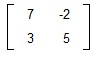Step a: Switch the elements on main diagonal would comprise switching the 5 and 7.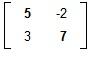Step b: Take the opposite of other two elements; however leave them where they are.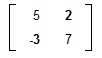Step c: Determine the determinant and divide each and every element by that. The determinant is the product of elements on the main diagonal minus the product of elements off the main diagonal. That signifies the determinant of this matrix is 7(5) - (-3)(2) = 35 + 6 = 41. We divide each and every element by 41.

The inverse of the original matrix is: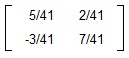It is stated that there was no matrix division. There is no division by the matrix. You might multiply or divide a matrix by a scalar (or real number) and the determinant is scalar.

Using the Calculator:

Now that you know how to determine the identity matrix by hand, let us talk practicality. The calculator will perform it for you.

Entering a Matrix:

a) Press the Matrix key (that is, right below the X key). On TI-83+, you will require to press the 2nd Matrix.

b) Arrow to the Edit sub-menu.

c) Select a Matrix to work with. You contain five to select from with the TI-82 and ten to select from with the TI-83. Usually, we will use [A]. Try to ignore using [E] for unspecified reasons which will be specified when you take Finite Mathematics.

d) Enter the number of rows, press enter and then enter the number of columns, followed by enter.

e) You now enter each and element in the matrix, reading from left to right and from top to bottom. Hit enter after each and every number. You might use the arrow keys to move around when you make a mistake.

f) Quit (2nd Mode) whenever you are completed entering all the numbers.

Using Matrices:

If you require to access the matrix that you have made, simply hit the Matrix key and select the suitable matrix. It is suggested that you begin by using Matrix 1, Matrix 2, and so on rather than Matrix, arrow down, enter. This will go faster and we will be doing a lot with such matrices.

Finding the Inverse of a Matrix on a Calculator
:

Enter the expression [A]-1 by going through Matrix 1, and then pressing the x-1 key. This will not work when you try to increase the matrix to -1 power as in [A]^(-1).

You might encompass to use the right or left arrow keys to scroll via the whole matrix to write it down. Please give precise answers if possible.

One way of providing precise answers is to have the calculator transform the decimals to fractions for you. We can have the calculator do decimal to fraction transformation by pressing Math, Enter, and Enter.

As well, when you get an answer such as 1.2E-12, chances are really excellent that number is zero and it is since of inaccuracies in the calculator that you are receiving that response. Transform the number to zero.

Why was it we required an inverse?

One of the major utilizations of inverses is to solve a system of the linear equations. We can write a system in matrix form as AX = B.

Now, pre-multiply both the sides by the inverse of A. Make sure that you meet such two conditions.

a) You should place the inverse of matrix adjacent to the matrix. That is as Inverses require to be next to one other (much loose mathematically, however think back to functions) in order to undo one other.

b) When you multiply by placing something in front of the left side (or pre-multiply), then it has to go in front of the right side. When you place something behind (or post-multiply) the left side, then it has to go behind the right side.

Matrix Multiplication is NOT Commutative!

A-1(AX) = A-1(B) ... pre-multiply both the sides by A-1
(A-1A) X = A-1 B ... utilize the associative property to re-group the factors.
I X = A-1 B ... whenever you multiply inverses altogether, then they become identity matrix.
X = A-1 B ... that is, the identity matrix is similar to multiplying by 1.
If AX = B, then X = A-1 B

Solving Systems of Linear Equations:

Let consider the system of linear equations:

3x + 2y - 5z = 12
x - 3y + 2z = -13
5x - y + 4z = 10

Write down the coefficients in an A matrix.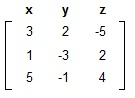Write down the variables in an X matrix.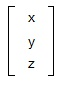Write down the constants in B matrix.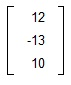Verify that AX = B:

This step is not really required; however I wanted to show you that this thing really does work.

AX will be a (3×3) × (3×1) = 3×1 matrix. The B matrix is also a 3×1 matrix; therefore at least the dimensions work out right.

Here is A times X.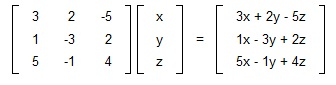Note that the turns out to be left side of the system of equations. The B is the right hand side, therefore we have achieved equality. We can write a system of the linear equations as AX = B.

Therefore, if you can write a system of linear equations as AX = B; here A is the coefficient matrix, then X is the variable matrix and B is the right hand side, we can find out the solution to the system by X = A-1 B.

Put the coefficient matrix to [A] on the calculator and the right hand side to [B].

Whenever you want the calculator to determine the inverse of coefficient matrix, then it would give you this for A-1.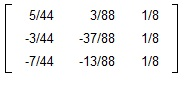We could do that and then multiply that by B; however it would be simpler just to place the whole expression to the calculator and get the outcome or answer directly. Even what is shown below is more work than is essential.

X = A-1 B = ….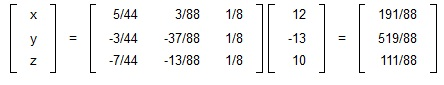There you go, x = 191/88, y = 519/88, and z = 111/88. That would be a real pain to resolve it by hand.

This is easy, why don't we always do this?

The major reason is as it does not always work.

a) Inverses only present for the square matrices. That signifies if you do not have same number of equations as variables, then you cannot use this technique.

b) Not all square matrix consists of an inverse. When the coefficient matrix A is singular (that is, has no inverse), then there might be no solution or there might be many solutions, however we cannot tell what it is.

c) Inverses are a pain to find out by hand. When you have a calculator, it is not so bad, however keep in mind that calculators do not always give you the answer you are looking for.

Latest technology based Algebra Online Tutoring Assistance

Tutors, at the www.tutorsglobe.com, take pledge to provide full satisfaction and assurance in Algebra help via online tutoring. Students are getting 100% satisfaction by online tutors across the globe. Here you can get homework help for Algebra, project ideas and tutorials. We provide email based Algebra help. You can join us to ask queries 24x7 with live, experienced and qualified online tutors specialized in Algebra. Through Online Tutoring, you would be able to complete your homework or assignments at your home. Tutors at the TutorsGlobe are committed to provide the best quality online tutoring assistance for Algebra Homework help and assignment help services. They use their experience, as they have solved thousands of the Algebra assignments, which may help you to solve your complex issues of Algebra. TutorsGlobe assure for the best quality compliance to your homework. Compromise with quality is not in our dictionary. If we feel that we are not able to provide the homework help as per the deadline or given instruction by the student, we refund the money of the student without any delay.